Courses

# General Introduction: Energy Methods in Structural Analysis - 2 Civil Engineering (CE) Notes | EduRev

## Civil Engineering (CE) : General Introduction: Energy Methods in Structural Analysis - 2 Civil Engineering (CE) Notes | EduRev

The document General Introduction: Energy Methods in Structural Analysis - 2 Civil Engineering (CE) Notes | EduRev is a part of the Civil Engineering (CE) Course Structural Analysis.
All you need of Civil Engineering (CE) at this link: Civil Engineering (CE)

Static Indeterminacy

The aim of structural analysis is to evaluate the external reactions, the deformed shape and internal stresses in the structure. If this can be accomplished by equations of equilibrium, then such structures are known as determinate structures. However, in many structures it is not possible to determine either reactions or internal stresses or both using equilibrium equations alone. Such structures are known as the statically indeterminate structures. The indeterminacy in a structure may be external, internal or both. A structure is said to be externally indeterminate if the number of reactions exceeds the number of equilibrium equations. Beams shown in Fig.1.8(a) and (b) have four reaction components, whereas we have only 3 equations of equilibrium. Hence the beams in Figs. 1.8(a) and (b) are externally indeterminate to the first degree. Similarly, the beam and frame shown in Figs. 1.8(c) and (d) are externally indeterminate to the 3rd degree.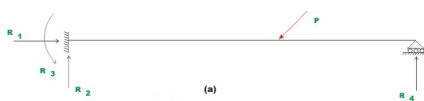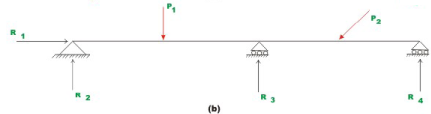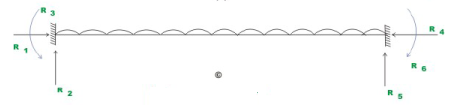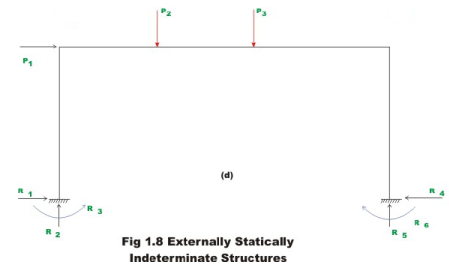Now, consider trusses shown in Figs. 1.9(a) and (b). In these structures, reactions could be evaluated based on the equations of equilibrium. However, member forces can not be determined based on statics alone. In Fig. 1.9(a), if one of the diagonal members is removed (cut) from the structure then the forces in the members can be calculated based on equations of equilibrium. Thus, structures shown in Figs. 1.9(a) and (b) are internally indeterminate to first degree.The truss and frame shown in Fig. 1.10(a) and (b) are both externally and internally indeterminate.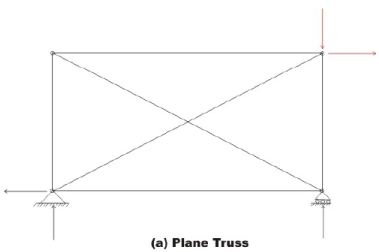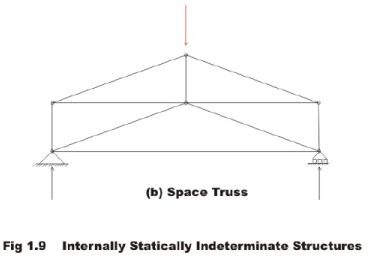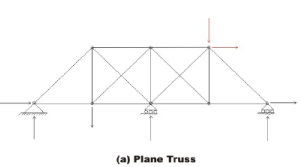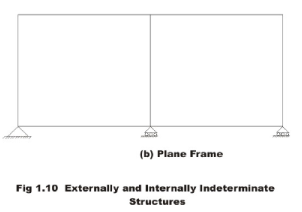So far, we have determined the degree of indeterminacy by inspection. Such an approach runs into difficulty when the number of members in a structure increases. Hence, let us derive an algebraic expression for calculating degree of static indeterminacy.

Consider a planar stable truss structure having m members and j joints. Let the number of unknown reaction components in the structure be r . Now, the total number of unknowns in the structure is m + r . At each joint we could write two equilibrium equations for planar truss structure,  viz.,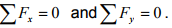Hence total number of equations that could be written is 2 j.

If 2 j = m + r then the structure is statically determinate as the number of unknowns are equal to the number of equations available to calculate them. The degree of indeterminacy may be calculated as

i = (m + r) - 2j           (1.4)

We could write similar expressions for space truss, plane frame, space frame and grillage. For example, the plane frame shown in Fig.1.11 (c) has 15 members, 12 joints and 9 reaction components. Hence, the degree of indeterminacy of the structure is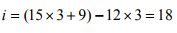Please note that here, at each joint we could write 3 equations of equilibrium for plane frame.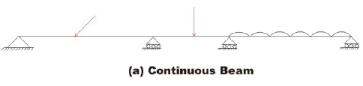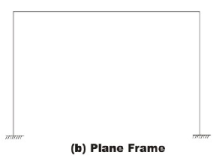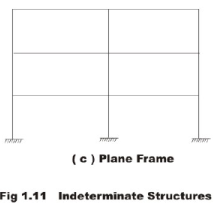Kinematic Indeterminacy

When the structure is loaded, the joints undergo displacements in the form of translations and rotations. In the displacement based analysis, these joint displacements are treated as unknown quantities. Consider a propped cantilever beam shown in Fig. 1.12 (a). Usually, the axial rigidity of the beam is so high that the change in its length along axial direction may be neglected. The displacements at a fixed support are zero. Hence, for a propped cantilever beam we have to evaluate only rotation at B and this is known as the kinematic indeterminacy of the structure. A fixed fixed beam is kinematically determinate but statically indeterminate to 3rd degree. A simply supported beam and a cantilever beam are kinematically indeterminate to 2nd degree.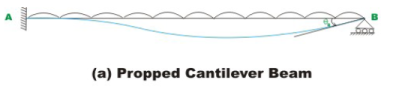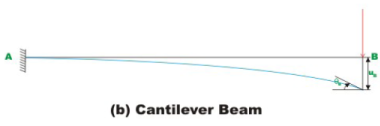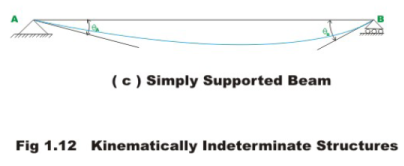The joint displacements in a structure is treated as independent if each displacement (translation and rotation) can be varied arbitrarily and independently of all other displacements. The number of independent joint displacement in a structure is known as the degree of kinematic indeterminacy or the number of degrees of freedom. In the plane frame shown in Fig. 1.13, the joints B and C have 3 degrees of freedom as shown in the figure. However if axial deformations of the members are neglected then u1 = u4 and u2 and u4 can be neglected. Hence, we have 3 independent joint displacement as shown in Fig. 1.13 i.e. rotations at B and C and one translation.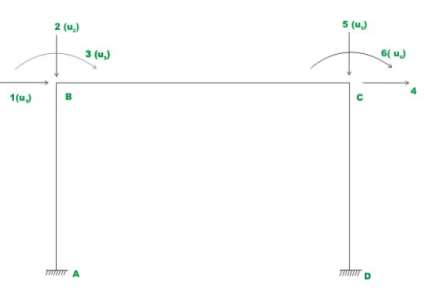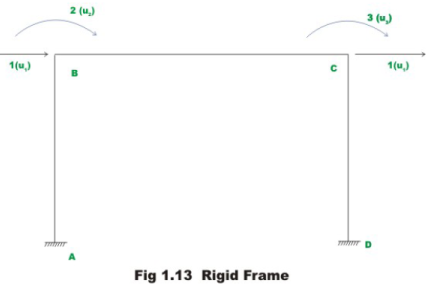Kinematically Unstable Structure

A beam which is supported on roller on both ends (vide. Fig. 1.14) on a horizontal surface can be in the state of static equilibrium only if the resultant of the system of applied loads is a vertical force or a couple. Although this beam is stable under special loading conditions, is unstable under a general type of loading conditions. When a system of forces whose resultant has a component in the horizontal direction is applied on this beam, the structure moves as a rigid body. Such structures are known as kinematically unstable structure. One should avoid such support conditions.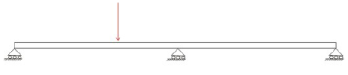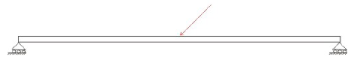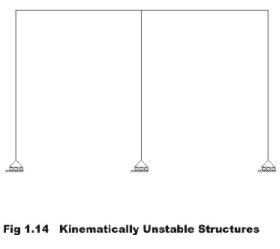Compatibility Equations

A structure apart from satisfying equilibrium conditions should also satisfy all the compatibility conditions. These conditions require that the displacements and rotations be continuous throughout the structure and compatible with the nature supports conditions. For example, at a fixed support this requires that displacement and slope should be zero.

Force-Displacement Relationship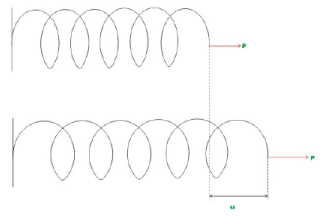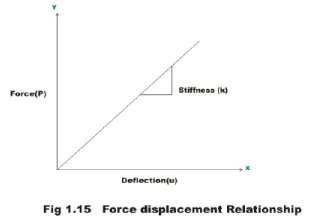Consider linear elastic spring as shown in Fig.1.15. Let us do a simple experiment. Apply a force P1 at the end of spring and measure the deformation u1. Now increase the load to P2 and measure the deformation u2. Likewise repeat the experiment for different values of load P1, P2,......Pn. Result may be represented in the form of a graph as shown in the above figure where load is shown on y-axis and deformation on abscissa. The slope of this graph is known as the stiffness of the spring and is represented by k and is given by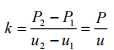(1.5)
P = ku      (1.6)

The spring stiffness may be defined as the force required for the unit deformation of the spring. The stiffness has a unit of force per unit elongation. The inverse of the stiffness is known as flexibility. It is usually denoted by a and it has a unit of displacement per unit force.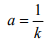(1.7)

the equation (1.6) may be written as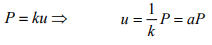(1.8)

The above relations discussed for linearly elastic spring will hold good for linearly elastic structures. As an example consider a simply supported beam subjected to a unit concentrated load at the centre. Now the deflection at the centre is given by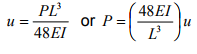(1.9)

The stiffness of a structure is defined as the force required for the unit deformation of the structure. Hence, the value of stiffness for the beam is equal to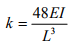As a second example, consider a cantilever beam subjected to a concentrated load (P) at its tip. Under the action of load, the beam deflects and from first principles the deflection below the load (u) may be calculated as,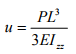(1.10)

For a given beam of constant cross section, length L , Young’s modulus E , and moment of inertia Izz the deflection is directly proportional to the applied load. The equation (1.10) may be written as

u = a P      (1.11)

Where a is the flexibility coefficient and is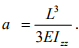Usually it is denoted by aij the flexibility coefficient at i due to unit force applied at j . Hence, the stiffness of the beam is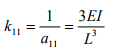(1.12)

Summary

In this lesson the structures are classified as: beams, plane truss, space truss, plane frame, space frame, arches, cables, plates and shell depending on how they support external load. The way in which the load is supported by each of these structural systems are discussed. Equations of static equilibrium have been stated with respect to planar and space and structures. A brief description of static indeterminacy and kinematic indeterminacy is explained with the help simple structural forms. The kinematically unstable structures are discussed in section 1.6. Compatibility equations and force-displacement relationships are discussed. The term stiffness and flexibility coefficients are defined. In section 1.8, the procedure to calculate stiffness of simple structure is discussed.

Offer running on EduRev: Apply code STAYHOME200 to get INR 200 off on our premium plan EduRev Infinity!

## Structural Analysis

30 videos|122 docs|28 tests

,

,

,

,

,

,

,

,

,

,

,

,

,

,

,

,

,

,

,

,

,

;